## Create Bell Curve Chart

The Bell curve chart is also called as normal probability distributions in Statistics and it will be used to show a plot of normal distribution of a given data set. This post will guide you how to create a chart of a bell curve in Microsoft Excel. And How to save the generated bell curve chart as normal chart template in Excel.

## Create Bell Curve Chart

You can refer to the below examples to create a sample bell curve, just do the following steps:

#1 enter the following column headings in a new worksheet (Data, Mean, Standard deviation, Distribution)

The mean is the average value of all the data points in range A2:A10. And the Standard Deviation indicates how much deviates from the mean of the data set.

#2 enter the data in the range A2:A10

#3 enter the formula =AVERAGE(A2:A10) in the formula box of cell B2 to generate the Mean value.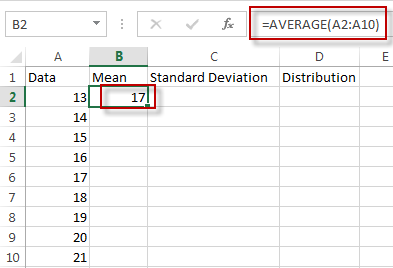#4 enter the formula =STDEV(A2:A10) in the formula box of cell C2 to generate the standard deviation.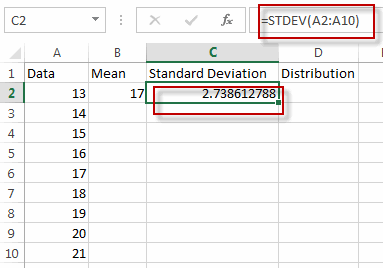#5 enter the formula =NORM.DIST(A2,\$B\$2,\$C\$2,FALSE) in the formula box of cell D2 to generate normal probability distribution. Then drag Auto Fill Handler over other cells from D2 to D10.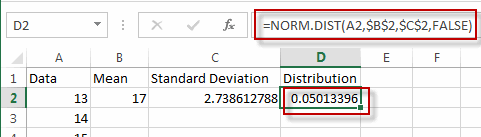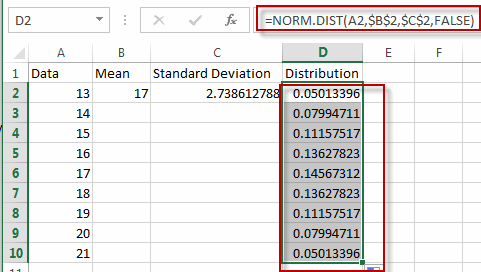#6 select range A2:A10 and D2:D10, then go to INSERT tab, click Scatter with Smooth Lines under charts group.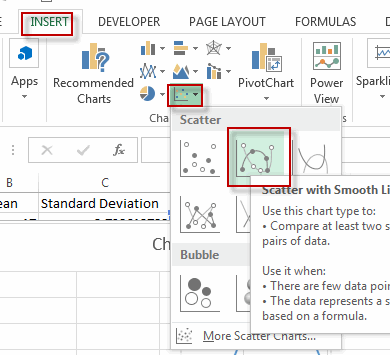#7 you will see a bell curve is created.

create bell curve chart5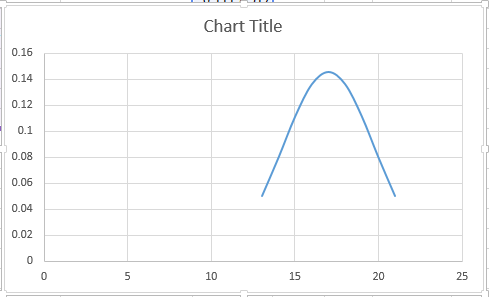## Save Bell Curve Chart as Chart Template

If you want to save the generated bell curve chart as chart template, just refer to the below steps:

#1 right click on the bell curve chart, select Save as Template… from the pop up menu list.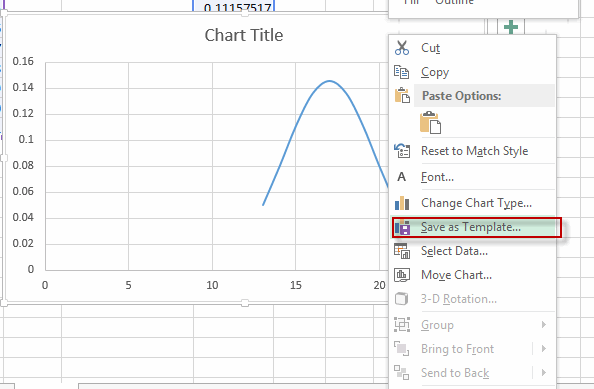#2 enter the file name, and click Save button.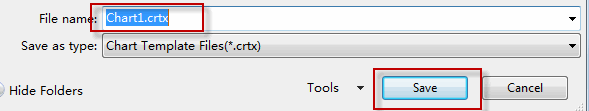### Related Functions

• Excel AVERAGE function
The Excel AVERAGE function returns the average of the numbers that you provided.The syntax of the AVERAGE function is as below:=AVERAGE (number1,[number2],…)….
• Excel STDEV Function
The Excel STDEV function returns the standard deviation of a population based on a sample of numbers. This function has been replaced by the STDEV.S function in Excel 2010.The syntax of the STDEV function is as below:= STDEV (number1,[number2],…)…

## Excel STDEV Function

This post will guide you how to use the STDEV function with syntax and examples in Microsoft excel.

### Description

The Excel STDEV function returns the standard deviation of a population based on a sample of numbers. This function has been replaced by the STDEV.S function in Excel 2010. The standard deviation is used to identify the amount that a set of values differ from the average value. And if you want to calculate the standard deviation for an entire population, you can use the STDEVP or STDEV.P function.

The STDEV function is a build-in function in Microsoft Excel and it is categorized as a Statistical Function.

The STDEV function is available in Excel 2016, Excel 2013, Excel 2010, Excel 2007, Excel 2003, Excel XP, Excel 2000, Excel 2011 for Mac.

### Syntax

The syntax of the STDEV function is as below:

`= STDEV (number1,[number2],...)`

Where the STDEV function arguments are:

• number1-This is a required argument.  The first number or cell reference in a sample of a population.
• number2 -This is an optional argument.  Number arguments 2 to 255 corresponding to a sample of a population. And it can only accept up to 30 number arguments in Excel 2003 or older.

Note:

• The argument must be numbers or arrays or cell references that contain only numbers.
• The STDEV function is only used for its arguments are a sample of the population. And if the argument represents the entire population, you need to use the STDEVP function to calculate the standard deviation.
• If the argument contain the logical values and text representations of numbers and those values will be counted.
• STDEV uses the following formula:

### Excel STDEV Function Examples

The below examples will show you how to use Excel STDEV Function to calculate the standard deviation for data that represents a sample of the population.

1# to get standard deviation of a sample of numbers, using the following formula:

`=STDEV(A1:A2)`

### Related Functions

• Excel STDEVA Function
The Excel STDEVA function returns the standard deviation of a population based on a sample of numbers, text, and logical values. The syntax of the STDEVA function is as below:= STDEVA (value1, [value2], ..)…
• Excel STDEVP Function
The Excel STDEVP function returns the standard deviation of a population based on an entire population of numbers. This function has been replaced by the STDEV.P function in Excel 2010.The syntax of the STDEVP function is as below:= STDEVP(number1,[number2],…)…
• Excel STDEVPA Function
The Excel STDEVPA function returns the standard deviation of a population based on an entire population of numbers, text or logical values. The syntax of the STDEVPA function is as below:= STDEVPA (value1, [value2], …)…